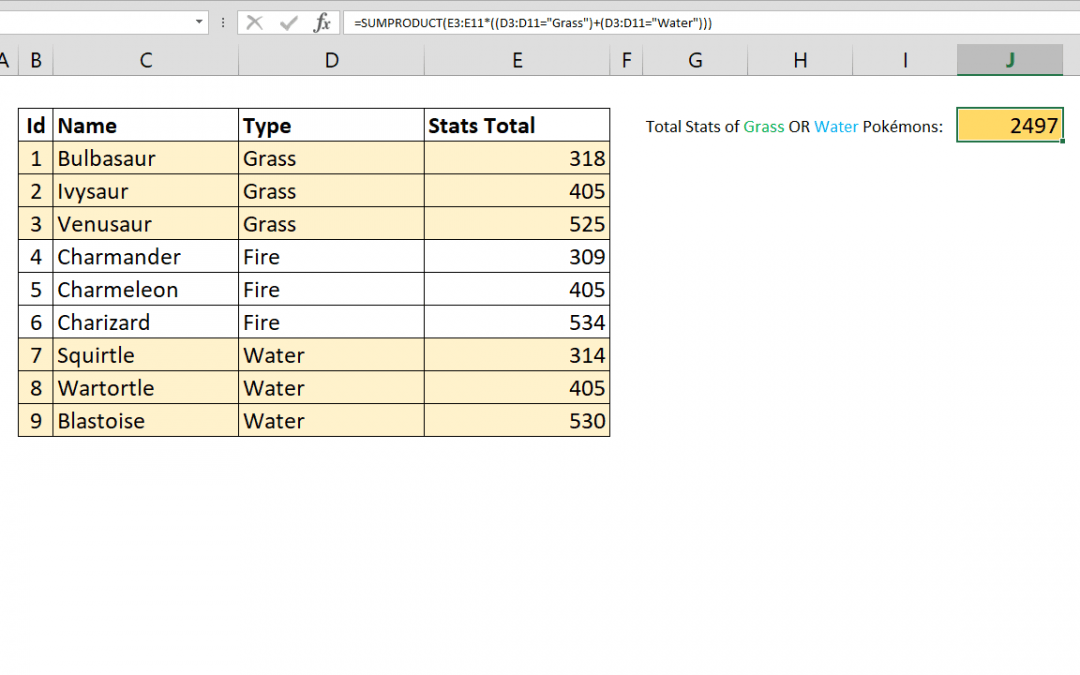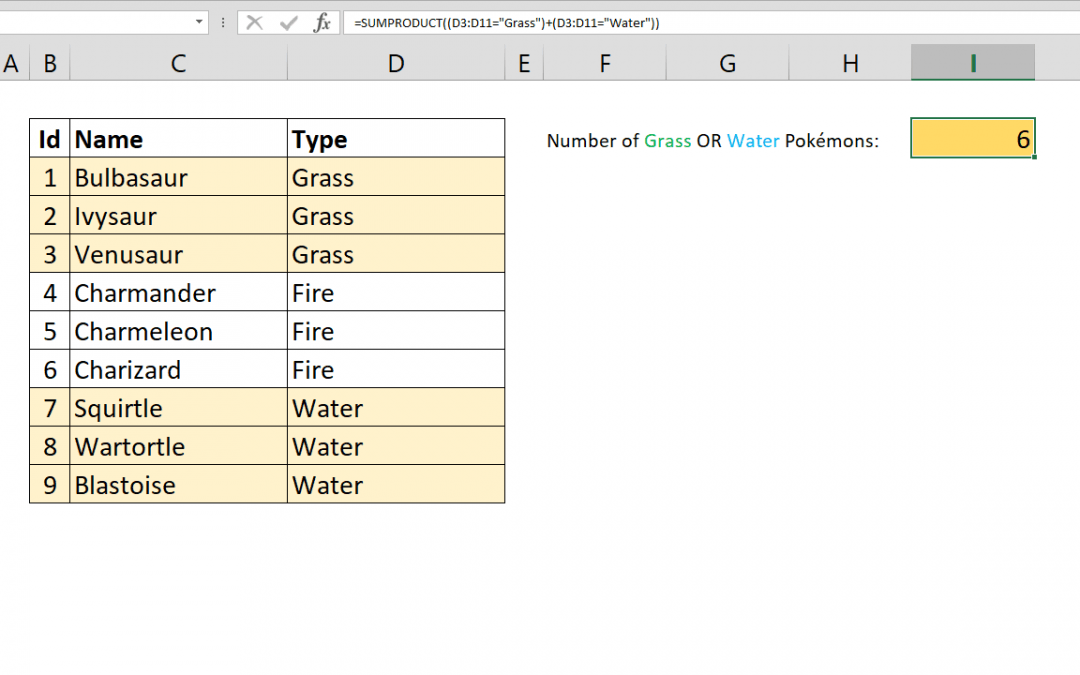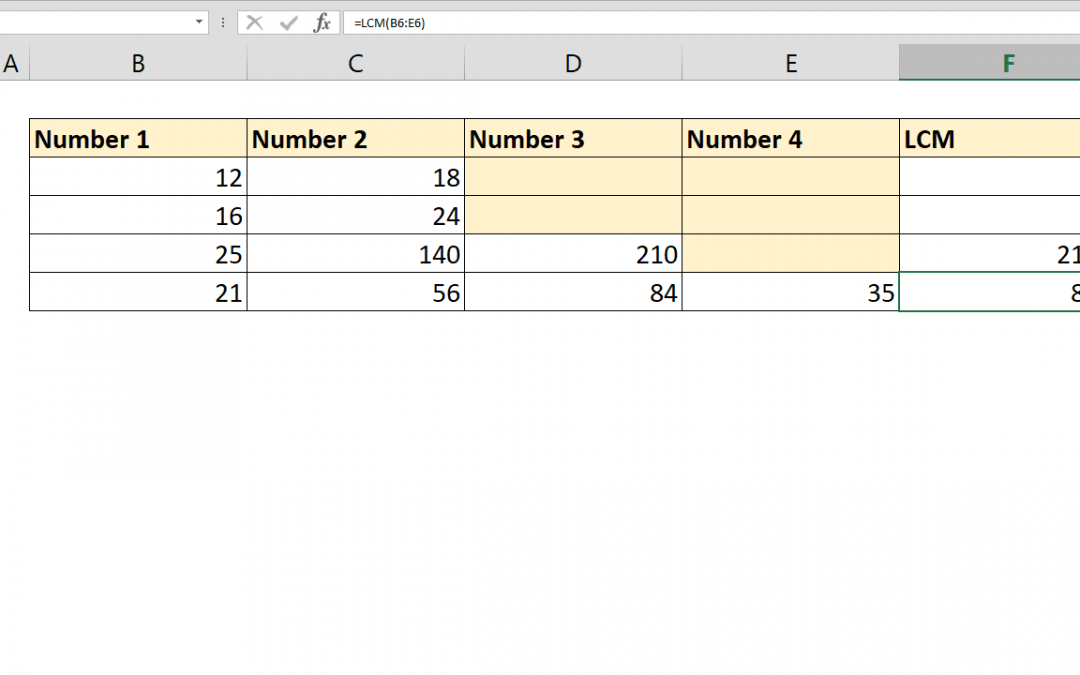Select Page## How to create a dynamic drop down list in Excel

Creating a dynamic Excel drop down list is very useful If you have a list that is updated frequently. This article shows you how to create a dynamic drop down list with the help of OFFSET and COUNTA functions. Syntax =OFFSET(title of list, 1, 0, COUNTA(column that...## How to get Excel cell address of a lookup result

This article shows you how to get Excel cell address of a lookup result by using the CELL and INDEX functions. Syntax =CELL( “address”, INDEX( data, MATCH( lookup value for row, lookup range for row, 0 ), column index ) ) Steps Start with the =CELL(...## How to change number format in Excel chart

Excel charts are one of the most used and easy to understand data visualization tools. Excel provides many chart types as well as numerous personalization options. One of the cool features is the ability to change number format in Excel chart. Steps Double-click on...## How to calculate average of values with OR operator

The AVERAGEIFS function can calculate the average of values with multiple conditions. However, these conditions are linked with AND operator. But if you want to handle AVERAGE OR combination, AVERAGEIFS function will not work. This article shows how to calculate...## How to sum values with OR operator using SUMPRODUCT with multiple criteria

The SUMIFS function can sum values based on multiple conditions. However, these conditions are all linked with AND operator. As a result, all conditions should be fulfilled to return a value. This article shows How you can sum values with multiple OR operators using...## How to count values with OR operator

You can count values in Excel by multiple criteria using the COUNTIFS function. However COUNTIFS will only link those criterion by AND operation. If you want to COUNTIFS OR, you need an alternative approach. This article shows how to count values with OR operator...## How to count characters in Excel in a range

The LEN function is used to count characters in Excel. But it doesn’t support ranges or arrays to easily count total number of characters in a range. This article shows 2 ways to count characters in Excel in a range which uses SUMPRODUCT and SUM along with LEN....## How to calculate Least Common Multiple

Excel has a built in function to calculate Least Common Multiple (LCM) of two or more integers. The function is also called LCM. Syntax =LCM(number1, [number2], …) Steps Start with =LCM( Type or select cells or range that includes integers B6:E6 Type ) to close the...